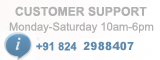# PIC Development Board

/*
* Project name:I2C
PIC Development Board
(c) Researchdesignlab.com
* Test configuration:
MCU: PIC16F877A
Dev.Board: PIC
Oscillator: 20.0 MHz
Software: MPLAB IDE v8.92(HI-TECH_C)
*/

#include<htc.h>
#include"string.h"
#include<stdio.h>
#define _XTAL_FREQ 20000000

#define I2C_FREQ 100 // 100khz at 4Mhz

#define FOSC 20000 // 20Mhz==>20000Khz

void WaitMSSP(void);

void i2c_init(void);

void I2C_Start(void);

void I2C_Stop(void);

char I2C_Write_Data(unsigned char);

void I2C_Reset(void);

void DelayMs(unsigned int);

char UART_Init(const long int);

void UART_Write_Text(char *);

void UART_Write(char );

void main()
{
char a;
UART_Init(9600); //initialize the UART
DelayMs(1000); //Provide a delay of 1s
i2c_init(); //initialize the I2C
DelayMs(1000); //Provide a delay of 1s

while(1)
{
I2C_Start(); //start bit is set in this function
DelayMs(100); //Provide a delay
I2C_Write_Data(0xa0); //write the data on to the location 0xa0(device address)
DelayMs(100); //Provide a delay
I2C_Write_Data(0x20); //write the data on to location 0x20
DelayMs(100); //Provide a delay
I2C_Write_Data('a'); //send character ‘a’
DelayMs(100); //Provide a delay
I2C_Stop(); //stop bit is set in this function
DelayMs(100); //Provide a delay
I2C_Start(); //start bit is set in this function
DelayMs(100); //Provide a delay
I2C_Write_Data(0xa0); //write the data on to the location 0xa0(device address)
DelayMs(100); //Provide a delay
I2C_Write_Data(0x20); //write the data on to location 0x20
DelayMs(100); //Provide a delay
I2C_Reset(); //this function is used to reset
DelayMs(100); //Provide a delay
I2C_Write_Data(0xa1); //write the data on to the location 0xa0(device address)
DelayMs(100); //Provide a delay
a=I2C_Read_Data(); //this function reads the data stored in EEPROM
UART_Write(a); //display the character on hyper terminal
DelayMs(100); //Provide a delay
I2C_Stop(); //stop bit is set in this function
DelayMs(100); //Provide a delay
}
}

char I2C_Write_Data(unsigned char data)//This function is used to write the data onto EEPROM

{

//WaitMSSP(); // wait for the operation to be finished

SSPBUF=data; //Send Slave address write command

WaitMSSP(); //wait for operation to complete
}

void I2C_Start() //this function is used to set start bit
{
SEN=1; //start bit is set
WaitMSSP(); //wait for operation to complete

}

void I2C_Stop() //this function is used to set start bit
{
PEN=1; //stop bit is set
WaitMSSP(); //wait for operation to complete
}

void I2C_Reset() //this function is used to reset start bit
{
RSEN=1; // Send re-start bit

WaitMSSP(); //wait for operation to complete

}
char I2C_Read_Data() //this function is used to read data from EEPROM

{

RCEN=1; // Enable receive

WaitMSSP(); //wait for operation to complete

ACKDT=1; // Acknowledge data 1: NACK, 0: ACK

ACKEN=1; // Enable ACK to send

WaitMSSP();//wait for operation to complete

return SSPBUF; // Send the received data to PC

DelayMs(30);

}

void WaitMSSP() // function for wait for operation to complete

{

while(!SSPIF); // while SSPIF=0 stay here else exit the loop

SSPIF=0; // operation completed clear the flag

}

void i2c_init() //function to initialize I2C
{

TRISC3=1; // Set up I2C lines by setting as input

TRISC4=1;

SSPCON=0x28; // SSP port, Master mode, clock = FOSC / (4 * (SSPADD+1))

SSPADD=(FOSC / (4 * I2C_FREQ)) - 1; //clock 100khz

SSPSTAT=80; // Slew rate control disabled

}

void DelayMs(unsigned int Ms) //function to provide a delay

{

int delay_cnst;

while(Ms>0)

{

Ms--;

for(delay_cnst = 0;delay_cnst <220;delay_cnst++);

}

}

char UART_Init(const long int baudrate)
{
unsigned int x;
x = (_XTAL_FREQ - baudrate*64)/(baudrate*64);
if(x>255)
{
x = (_XTAL_FREQ - baudrate*16)/(baudrate*16);
BRGH = 1; //High Baud Rate Select bit set to high
}
if(x<256)
{
SPBRG = x; //Writing SPBRG register
SYNC = 0; //Selecting Asynchronous Mode
SPEN = 1; //enables serial port
TRISC7 = 1;
TRISC6 = 1;
CREN = 1; //enables continuous reception
TXEN = 1; //enables continuous transmission
return 1;
}
return 0;
}

char UART_TX_Empty()
{
return TRMT; //Returns Transmit Shift Status bit
}

{
return RCIF; //Flag bit
}

char UART_Read() //this function is used to read a byte
{
while(!RCIF); //Waits for Reception to complete
return RCREG; //Returns the 8 bit data
}
void UART_Read_Text(char *Output, unsigned int length)//this function is used to read a text
{
int i;
for(int i=0;i<length;i++)
}

void UART_Write(char data) //this function is used to write a byte
{
while(!TRMT);
TXREG = data; //transmit register
}

void UART_Write_Text(char *text) //this function is used to write a string
{
int i;
for(i=0;text[i]!='\0';i++)
UART_Write(text[i]);
}